×#### Thank you for registering.

One of our academic counsellors will contact you within 1 working day.

Click to Chat

1800-1023-196

+91-120-4616500

CART 0

• 0

MY CART (5)

Use Coupon: CART20 and get 20% off on all online Study Material

ITEM
DETAILS
MRP
DISCOUNT
FINAL PRICE
Total Price: Rs.

There are no items in this cart.
Continue Shopping```Area Enclosed Between the CurvesArea between curves y = Φ(x) and y = Ψ(x) and ordinates x = x1 and x = x2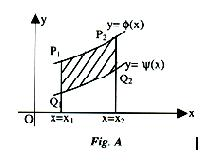(i) To determine the area between curves, first find out the points of intersection of the two curves. (See figure)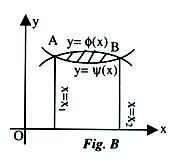(ii) If in the domain common to both (i.e. the domain given by the points of intersection) the curves lie above x-axis, then area is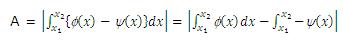Note: If however one part of one or both the curve lies below x-axis, then the individual integral must be evaluated according to the case considered in the last topic.

3. Area between curve y = f(x) and y-axis.
To obtain the area between the curve and the y-axis, the function must be written in y. (see figure A and B) i.e. y = f(x) must be inverted to x = g(y) (where g(x) = f–1 (x)) and the integral to be evaluated is ∫y1y2x dy or ∫y1y2g(y) dy.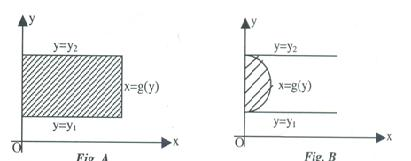Similarly the area bounded between the y-axis & the curves y = f(x) and y = g(x) can be determined. In general, to find the area of the region one must draw the curve and locate the region. The limits and sign of different definite integral are determined accordingly.

Illustration:
Suppose we have to calculate the area bounded by y2 = 4x and 2x = y.
Solution:
First we should calculate the points of intersection.
4x2 – 4x = 0   ⇒ x = 0, x = 1The shaded portion gives the required area.
This area is given by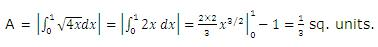Illustration:
Find the area bounded by y = x |sin x| and the x-axis between x = 0, x = 2Π. Solution: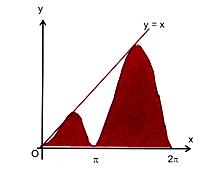Hence the required area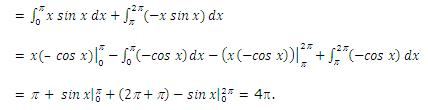Illustration:
Find the area bounded by the curve |x| + y = 1 and the x-axis.
Solution:
The given curve is |x| + y = 1 …… (1)
i.e. x + y = 1, when x > 0
and –x + y = 1, when x < 0.The required area
= area (CAOC) + area (OABO)= 1 sq. unit.
Note:    Obviously, y = 1 – [x] is an even function. Hence graph of y = 1 – |x| is symmetrical about the y-axis. Thus the required area = 2 ∫01(1-x)dx.

Illustration:
Calculate the area bounded by the curve y = x(3 – x)2, the x-axis and the ordinates of the maximum and minimum points of the curve.
Solution:
Since y = x(3 – x)2. Now for points of maxima or minima, we have dy/dx = 0
⇒ (3 – x)2 – 2x(3 – x) = 0
⇒ (3 – x)(3 – x – 2x) = 0          ∴ x = 1, 3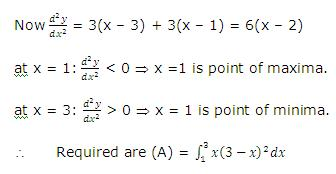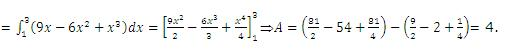Illustration:
Let f be a real valued function satisfying f(x/y) = f(x) – f(y) and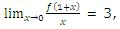then find the area bounded by y = f(x), y-axis and line y = 3.
Solution:
f(x/y) = f(x) – f(y)
Putting x = y = 1, we get f(1) = 0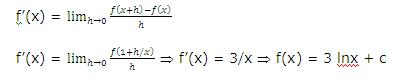Putting x = 1, we get c = 0
f(x) = 3 lnx
Hence required area = ∫∞3 e y/3 dy = 3e sq. units.

Illustration:
Find the area between curves y = exlnx and y = lnx/ex.
Solution:
The two curves intersect where⇒ x = 1/e or x = 1 (lnx is not defined for x = –1/e)
At x = 1/e or ex = 1
lnx = –1, y = –1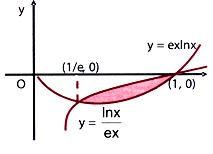⇒ (1/e, –1) is one point of intersection and at x = 1, ln 1 = 0, y = 0
⇒ (1, 0) is the other common point of the curves.
The required area = ∫1/e1  (y1-y2) dxIllustration:
Let f(x) = minimum (x + 1, √(1-x)) for all x < 1. Find the area bounded by y = f(x) and the x-axis.
Solution:
Required area = Area ABCA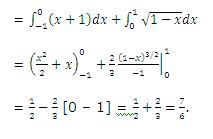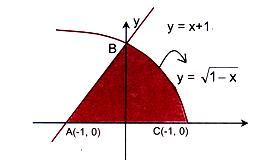To read more, Buy study materials of Application of Integration comprising study notes, revision notes, video lectures, previous year solved questions etc. Also browse for more study materials on Mathematics here.
```### Course Features

• 731 Video Lectures
• Revision Notes
• Previous Year Papers
• Mind Map
• Study Planner
• NCERT Solutions
• Discussion Forum
• Test paper with Video Solution